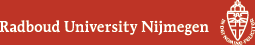## Geometry Seminar - Abstracts

### Talk

Wednesday 14 November 2018, 14:00-15:00 in HG03.085
Alex Berkovich (Gainesville, Florida)
Sudler's products revisited

### Abstract

Let $$(1-q)(1-q^2)\cdots(1-q^m) = \sum_{n\ge0} c_m(n)q^n$$. In this talk I discuss how to use $$q$$-binomial theorem together with the Euler pentagonal number theorem to show that $$\max_{n\ge0}|c_m(n)|=1$$ iff $$m = 0, 1, 2, 3, 5$$. There are many other similar results. For example, it can be proven that no positive integer $$m$$ exists, such that $$\max_{n\ge0}|c_m(n)|=9$$. The talk is based on a joint work with Ali K. Uncu (RISC, Linz, Austria).

(Back to geometry seminar schedule)